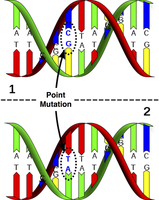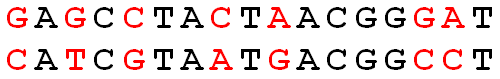# Counting Point Mutations solved by 27241

July 2, 2012, midnight by Rosalind Team

Topics: Alignment

## Evolution as a Sequence of MistakesFigure 1. A point mutation in DNA changing a C-G pair to an A-T pair.

A mutation is simply a mistake that occurs during the creation or copying of a nucleic acid, in particular DNA. Because nucleic acids are vital to cellular functions, mutations tend to cause a ripple effect throughout the cell. Although mutations are technically mistakes, a very rare mutation may equip the cell with a beneficial attribute. In fact, the macro effects of evolution are attributable by the accumulated result of beneficial microscopic mutations over many generations.

The simplest and most common type of nucleic acid mutation is a point mutation, which replaces one base with another at a single nucleotide. In the case of DNA, a point mutation must change the complementary base accordingly; see Figure 1.

Two DNA strands taken from different organism or species genomes are homologous if they share a recent ancestor; thus, counting the number of bases at which homologous strands differ provides us with the minimum number of point mutations that could have occurred on the evolutionary path between the two strands.

We are interested in minimizing the number of (point) mutations separating two species because of the biological principle of parsimony, which demands that evolutionary histories should be as simply explained as possible.

## ProblemFigure 2. The Hamming distance between these two strings is 7. Mismatched symbols are colored red.

Given two strings $s$ and $t$ of equal length, the Hamming distance between $s$ and $t$, denoted $d_{\mathrm{H}}(s, t)$, is the number of corresponding symbols that differ in $s$ and $t$. See Figure 2.

Given: Two DNA strings $s$ and $t$ of equal length (not exceeding 1 kbp).

Return: The Hamming distance $d_{\mathrm{H}}(s, t)$.

## Sample Dataset

GAGCCTACTAACGGGAT
CATCGTAATGACGGCCT


## Sample Output

7